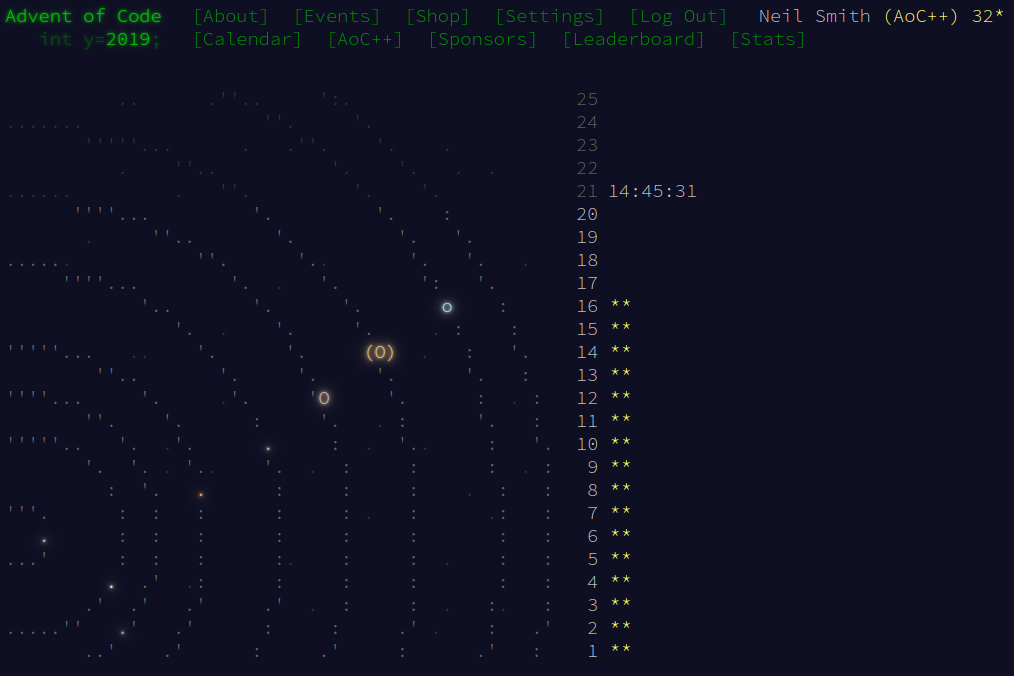December 20, 2019

# Advent of Code 2019 day 16

Exploiting built-in functions, and some special-case optimisation.Day 16 fits very nicely with the sorts of data handling functions built into the Haskell standard library.

## Part 1

I built this bottom-up, writing functions to do the tasks I needed to complete the specification. There wasn't a great deal of planning or deep thought involved in this.

I can expand the given pattern by using replicate, and using map to apply it to all the digits in the base pattern. That gives me a list of lists of digits, so I flatten it by using concatMap. cycle turns the expaned pattern into an infinite list, and I drop the first digit from it.

basePattern :: [Int]
basePattern = [0, 1, 0, -1]

patternOf :: Int -> [Int]
patternOf index = drop 1 $cycle$ concatMap (replicate index) basePattern


I can combine the digits and the expanded pattern to find the new digit at each index. I do that by merging the digits and the pattern, combining elements with (*), and summing the result (mod 10). The abs is there in case the sum is negative.

elementAt :: [Int] -> Int -> Int
elementAt digits index = (abs $sum$ zipWith (*) digits $patternOf index) mod 10  A single FFT round comprises finding the element at each index. The full FFT sequence is an iterateion of the FFT rounds. fftStep :: [Int] -> [Int] fftStep digits = map (elementAt digits) [1..(length digits)] fft :: [Int] -> [[Int]] fft = iterate fftStep  The solution is picking out the 101st value returned by fft, taking the first 8 digits, and formatting it for a neater display. part1 :: [Int] -> String part1 digits = map intToDigit$ take 8 $(fft digits)!!100  ## Part 2 This was a situation where brute force wasn't going to solve the problem. The naive approach (below) took far too long. part2naive :: [Int] -> String part2naive digits = map intToDigit signal where fullDigits = concat$ replicate 10000 digits
result = (fft fullDigits)!!100
offset = read @Int $map intToDigit$ take 7 digits
signal = take 8 $drop offset result  ### Aside: visible type application The code snippet above uses the @Int notation to tell read what type it should return. This is an example of the visible type applications extension to GHC. It certainly makes this bit of code neater than trying to give a full type definition in the middle of things! ### Going faster The trick is to exploit the structure of the extended pattern, especially when applied to the last few digits of the message. When we're calculating the last digit in the new message, the pattern for that new digit will be many zeros and one one: it will look like [0, 0 … 0, 1]. That means the last digit of the message remains the same. The pattern for the second-from-last digit is [0, 0 … 0, 1, 1], so the second-from-last digit in the new message is the sum of the last two digits in the old message (mod 10). The third-from-last digit uses the pattern s [0, 0 … 0, 1, 1, 1], so it's the sum of the last three digits in the old message (mod 10). This structure repeats for all the digits in the latter half of the message; with earlier digits, the pattern will start to look like [0, 0 … 0, 1, 1 … 0]. But that's not a problem in this case, as the offset means we only need the last 6000 or so digits in the 6,500,000-digit message. That gives an obvious implementation. The last digit is the sum of the last one digits; the second-from-last digit is the sum of the last two digits; and so on.The function tails gives me a list of the suffixes of the list, and in the order I want. fastFftStep :: [Int] -> [Int] fastFftStep digits = map (\ds -> (sum ds) mod 10)$ tails digits


Unfortunately, that's not quick enough.

But there's an even faster way, as clearly outlined by paul2718 on Reddit. The second-from-last digit in the new message is the last digit in the new message plus the second-from-last digit in the old message (mod 10). The third-from-last digit in the new message is the second-from-last digit in the new message plus the third-from-last digit in the old message.

In other words, a message that ends abcdef will become a message that ends ghijkl, like this:

a+b+c+d+e+f = g = a+h
b+c+d+e+f = h = b+i
c+d+e+f = i = c+j
d+e+f = j = d+k
e+f = k = e+l
f = l


scanr does that repeated summing for me, and keeps all the results in a list.

fastFftStep digits = scanr (\d s -> (d + s) mod 10) 0 digits


And that's it!

Code

The code is available, and on Github.